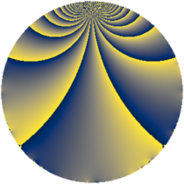# Properties

 Label 605.2.bLevel $605$ Weight $2$ Character orbit 605.b Rep. character $\chi_{605}(364,\cdot)$ Character field $\Q$ Dimension $46$ Newform subspaces $8$ Sturm bound $132$ Trace bound $6$

# Related objects

## Defining parameters

 Level: $$N$$ $$=$$ $$605 = 5 \cdot 11^{2}$$ Weight: $$k$$ $$=$$ $$2$$ Character orbit: $$[\chi]$$ $$=$$ 605.b (of order $$2$$ and degree $$1$$) Character conductor: $$\operatorname{cond}(\chi)$$ $$=$$ $$5$$ Character field: $$\Q$$ Newform subspaces: $$8$$ Sturm bound: $$132$$ Trace bound: $$6$$ Distinguishing $$T_p$$: $$2$$, $$19$$

## Dimensions

The following table gives the dimensions of various subspaces of $$M_{2}(605, [\chi])$$.

Total New Old
Modular forms 78 64 14
Cusp forms 54 46 8
Eisenstein series 24 18 6

## Trace form

 $$46q - 34q^{4} + 2q^{5} - 8q^{6} - 26q^{9} + O(q^{10})$$ $$46q - 34q^{4} + 2q^{5} - 8q^{6} - 26q^{9} + 10q^{10} - 16q^{14} + 2q^{15} + 10q^{16} + 16q^{19} + 16q^{20} - 12q^{21} - 4q^{24} + 2q^{25} + 44q^{26} + 12q^{29} + 6q^{30} - 8q^{31} - 40q^{34} - 18q^{35} + 22q^{36} - 28q^{40} - 12q^{41} - 36q^{45} + 8q^{46} + 30q^{49} + 18q^{50} + 28q^{51} - 20q^{54} + 52q^{56} - 24q^{59} - 40q^{60} - 20q^{61} + 38q^{64} - 4q^{69} - 8q^{70} + 24q^{71} - 12q^{74} + 26q^{75} - 24q^{76} + 28q^{79} - 56q^{80} - 18q^{81} - 48q^{84} - 2q^{85} - 4q^{86} - 36q^{89} + 28q^{90} - 8q^{91} + 44q^{94} - 12q^{95} - 36q^{96} + O(q^{100})$$

## Decomposition of $$S_{2}^{\mathrm{new}}(605, [\chi])$$ into newform subspaces

Label Dim. $$A$$ Field CM Traces $q$-expansion
$$a_2$$ $$a_3$$ $$a_5$$ $$a_7$$
605.2.b.a $$2$$ $$4.831$$ $$\Q(\sqrt{-11})$$ $$\Q(\sqrt{-11})$$ $$0$$ $$0$$ $$3$$ $$0$$ $$q+(-1+2\beta )q^{3}+2q^{4}+(1+\beta )q^{5}+\cdots$$
605.2.b.b $$4$$ $$4.831$$ 4.0.4400.1 $$\Q(\sqrt{-55})$$ $$0$$ $$0$$ $$0$$ $$0$$ $$q-\beta _{2}q^{2}+(-2-\beta _{3})q^{4}+\beta _{3}q^{5}-\beta _{1}q^{7}+\cdots$$
605.2.b.c $$4$$ $$4.831$$ $$\Q(\sqrt{-3}, \sqrt{-11})$$ None $$0$$ $$0$$ $$-3$$ $$0$$ $$q+(-\beta _{1}-\beta _{2}+\beta _{3})q^{2}+(-\beta _{1}+\beta _{3})q^{3}+\cdots$$
605.2.b.d $$4$$ $$4.831$$ $$\Q(\sqrt{-2}, \sqrt{3})$$ None $$0$$ $$0$$ $$0$$ $$0$$ $$q+\beta _{1}q^{2}+\beta _{3}q^{3}+\beta _{2}q^{4}+(\beta _{1}+\beta _{2}+\cdots)q^{5}+\cdots$$
605.2.b.e $$4$$ $$4.831$$ $$\Q(\sqrt{-2}, \sqrt{3})$$ None $$0$$ $$0$$ $$0$$ $$0$$ $$q+\beta _{1}q^{2}-\beta _{3}q^{3}+\beta _{2}q^{4}+(-\beta _{1}+\beta _{2}+\cdots)q^{5}+\cdots$$
605.2.b.f $$8$$ $$4.831$$ 8.0.1480160000.1 None $$0$$ $$0$$ $$4$$ $$0$$ $$q+\beta _{1}q^{2}+(\beta _{1}-\beta _{6}+\beta _{7})q^{3}+\beta _{2}q^{4}+\cdots$$
605.2.b.g $$8$$ $$4.831$$ 8.0.1480160000.1 None $$0$$ $$0$$ $$4$$ $$0$$ $$q+\beta _{1}q^{2}+(-\beta _{1}+\beta _{6}-\beta _{7})q^{3}+\beta _{2}q^{4}+\cdots$$
605.2.b.h $$12$$ $$4.831$$ $$\mathbb{Q}[x]/(x^{12} - \cdots)$$ None $$0$$ $$0$$ $$-6$$ $$0$$ $$q+\beta _{9}q^{2}-\beta _{8}q^{3}+(-2-\beta _{6})q^{4}+\beta _{4}q^{5}+\cdots$$

## Decomposition of $$S_{2}^{\mathrm{old}}(605, [\chi])$$ into lower level spaces

$$S_{2}^{\mathrm{old}}(605, [\chi]) \cong$$ $$S_{2}^{\mathrm{new}}(55, [\chi])$$$$^{\oplus 2}$$